Intoduction

Feature strength in Random Forests is difficult to assess. In their paper, “High-dimensional variable selection for survival data”, Ishwaran, Hemant, et al. describe a helpful metric; the minimal depth at which a variable is used to split, on average, in the forest . The climbeR package is intended to calculate and visualize this metric, given the result of a Random Forest experiment created with the R Ranger package.

To demonstrate climbeR, we need the results of a Random Forest experiment. For this example, we’ll be using Ranger’s Random Forest algorithm on the Iris and Veteran data sets, which will produce forests that we can climb (The verb climb, and the title of this package, are an analogy for the tree traversal necessary to calculate minimal depth of a maximal subtree).

Iris Classification Feature Strength

To illustrate a simple application of the climbeR package we’ll be using the results of a Ranger classifictaion model on the Iris data set.

Make a Random Forest

To start, lets make our forest. The following code is taken from the examples section of the Ranger documentation.

require(ranger)
## Classification forest with default settings
ranger(Species ~ ., data = iris)
## Ranger result
##
## Call:
##  ranger(Species ~ ., data = iris)
##
## Type:                             Classification
## Number of trees:                  500
## Sample size:                      150
## Number of independent variables:  4
## Mtry:                             2
## Target node size:                 1
## Variable importance mode:         none
## OOB prediction error:             5.33 %
## Prediction
train.idx <- sample(nrow(iris), 2/3 * nrow(iris))
iris.train <- iris[train.idx, ]
iris.test <- iris[-train.idx, ]
rg.iris <- ranger(Species ~ ., data = iris.train, write.forest = TRUE, importance = "impurity", num.trees = 2000)
pred.iris <- predict(rg.iris, dat = iris.test)
table(iris.test$Species, pred.iris$predictions)
##
##              setosa versicolor virginica
##   setosa         17          0         0
##   versicolor      0         15         4
##   virginica       0          0        14

Notice that Ranger has a built-in feature strength functionality - set with the importance argument - which can be turned on while running the experiment. Climber will offer an alternative to this VIMP Variable Importance, which can be calculated after the experiment is run, when the Random Forest has already been generated. The Ranger result rg.iris will have the property rg.iris$forest that can be passed to climbeR. This snapshot of the experiment’s random forest will allow climbeR to calculate minimal depth of maximal subtrees. Using climbeR The top level function in climbeR is getAndPlotMetric, which calculates and plots the metric (minimal depth of a maximal subtree). The function generates two plots of the metric, which offer different insights. The first is “Second Order vs First Order Minimal Depth of a Maximal Subtree”. “First Order” is the metric, as described above, and “Second Order” is the second most minimal depth of a maximal subtree. When a feature is used to split at a certain depth, it can occur in multiple maximal subtrees at that depth, or it can be split on at lower depths, as well. The depth of the second least deep subtree is called the “Second Order Minimal Depth of a Maximal Subtree”. Second order depth can reinforce our confidence in a feature’s importance. Strong features with large amounts of predictive information will have high first and second order values for the metric. library(climbeR) # call to climber function result <- getAndPlotMetric(rg.iris) # ^ evaluated data ^ eval_data <- result$subtree_metrics
# second order vs first order plot
so_vs_fo <- result$so_vs_fo_plot # number of splits vs first order plot ns_vs_fo <- result$ns_vs_fo

Lets take a look at the $so_vs_fo (Second Order vs First Order) property of the result object created above. # let's take a look plot(so_vs_fo)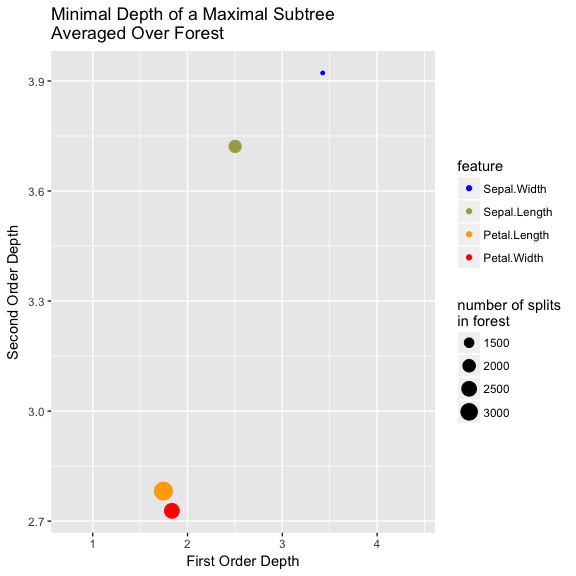The metric’s high scoring features will appear closest to the bottom left corner of the plot. How does this feature strength metric compare to the VIMP results of ranger? knitr::kable(combined_scores) first_order second_order normalized_counts counts feature VIMP 1.745754 2.782030 0.3825770 3465 Petal.Length 31.819771 1.835315 2.728045 0.2787899 2525 Petal.Width 25.235833 2.502388 3.721519 0.2161864 1958 Sepal.Length 7.664203 3.425968 3.921875 0.1224467 1109 Sepal.Width 1.101459 In the plot above, dot size represents split frequency of the feature in the forest. A Boolean variable can only be used to split once per population. This effect limits split frequency (choosing to split on a Boolean feature prevents descendant nodes from using that feature). A variable with high cardinality can be used to split many times per population, which can have the effect of saturating a tree’s decision nodes, and inflating the average minimal depth of a maximal subtree. This means that the metric weighs variables of disparate cardinalities unfairly. To further illustrate this bias towards features with high cardinality, we can plot the metric against split frequency. # second order vs first order plot plot(result$ns_vs_fo)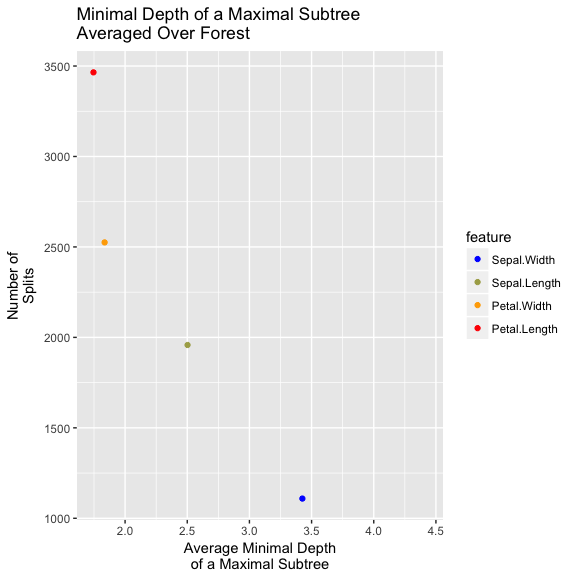You’ll note that high scoring features also tend to have high cardinality (floats, ints, features with many categories). We can also look at the raw data for another perspective:

# call to climber function
result <- getAndPlotMetric(rg.iris)
# evaluated data

The output of the call to ranger has a property rg.veteran$forest. This is a snapshot of the Random Survival Forest created by Ranger. ClimbeR can also evaluate these kinds of forests. Using climbeR Now that we have the result of a Random Survival Forest experiment, we can feed it to climbeR. library(climbeR) # call to climber function result <- getAndPlotMetric(rg.veteran) # evaluated data eval_data <- result$subtree_metrics
# second ord. vs first ord. plot
so_vs_fo <- result$so_vs_fo_plot Lets take at the$so_vs_fo property of the result created above.

# let's take a look
plot(so_vs_fo)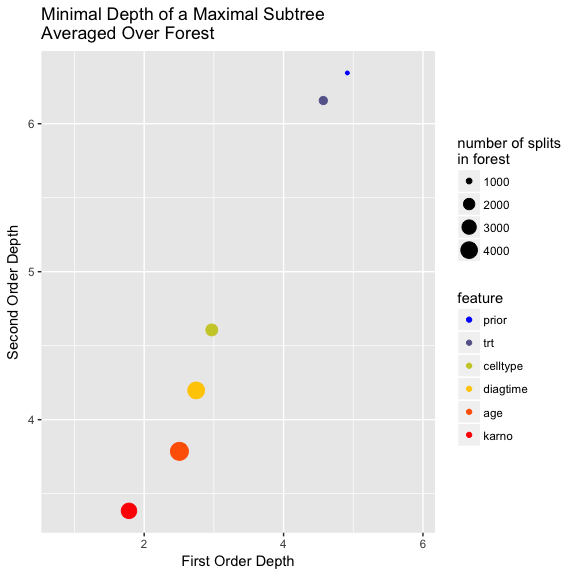It is important to note that although a variable has a large value for average minimal depth (occurs in the top right corner), it may still be an important variable for the Random Forest. The subtree depth metric (as well as Random Forests in general) is biased towards continuous variables. For example, in the plot above, celltype probably has a larger first and second order depth than karno and age. Karno and age are indeed predictive covariates, but they have high cardinality - so the metric will be biased towards them. In reality celltype has significant predictive information, but because it is a categorical variable with low cardinality, it is not scored highly by this metric.

Karno and age, which have high cardinality, have larger points than celltype, prior or trt variables, which are categorical covariates with low cardinality. The metric is biased towards these high cardinality features.

We can also look at the results data in table form:

# another look at the result
knitr::kable(eval_data)
first_order second_order normalized_counts counts
karno 1.782000 3.384000 0.2086595 3465
age 2.506000 3.786000 0.2829700 4699
diagtime 2.746000 4.198000 0.2447308 4064
celltype 2.970000 4.606426 0.1289895 2142
trt 4.570248 6.156716 0.0784656 1303
prior 4.915179 6.343137 0.0561845 933

Evaluating with Noise Features

For more insight into the metric’s bias towards continuous variables, we can add continuous noise to our data set, and see if the metric helps us distinguish between noise and real features.

Noise Generation

First, lets make some noise. We’ll define a simple function for adding n continuous or discrete noise covariates to a data set:

# this function generates noise features
addNoise <- function(input_df, n, discrete = FALSE){
df.len <- length(input_df[])
for(i in 1:n){
#add a categorical noise variable w/ cardinality: 4
noise_vect <- rnorm(df.len)
if(discrete){
#create RV w/ cardinality 4 (same as celltype)
noise_vect <- sample(c(0, 1, 2, 3), df.len, replace = TRUE)
}
name <- paste0("n_", toString(i))
input_df <- input_df %>% mutate(noise_vect)
names(input_df)[length(input_df)] <- name
}
return(input_df)
}

Evaluate Metric with Continuous Noise

Now we’re ready to add some noise and rerun the experiment.

# make some noise!
veteran <- addNoise(veteran, 5)

# rerun RSF (ranger), on the veteran data set
rg.veteran <- ranger(Surv(time, status) ~ .,
data = veteran,
write.forest = TRUE)

# call to climber function
result <- getAndPlotMetric(rg.veteran)
# ^ evaluated data ^
eval_data <- result$subtree_metrics # second ord. vs first ord. plot so_vs_fo <- result$so_vs_fo_plot

Lets take at the plot:

# let's take a look
plot(so_vs_fo)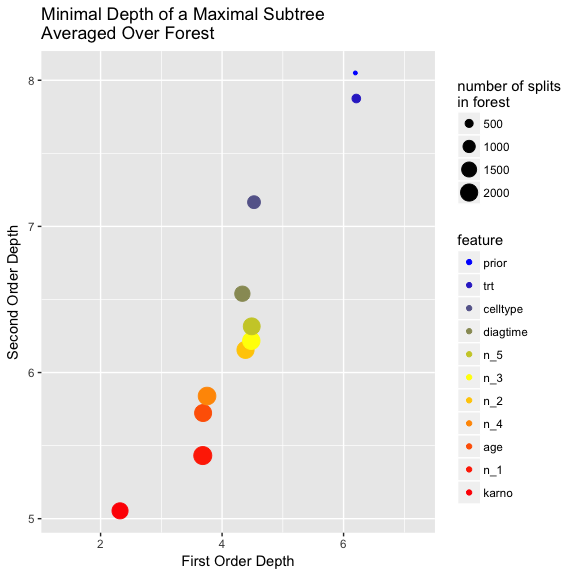The noise variables are labeled n_#, 1 through 5. How do the continuous noise variables rank, compared to the categorical variables? They probably beat celltype, which is bad because we know the noise features contain no information! This is why minimal depth of a maximal subtree is not a robust or perfect feature strength method.

Evaluate Metric with Discrete Noise

To push the analysis a little further, we can see if the metric helps us distinguish between the predictive categorical variables and some categorical variables generated from noise. We’ll remove the continuous noise features just to keep the plot uncluttered, and then add 5 discrete noise features, each with cardinality 4.

# just removing the noise features by reloading the data set
data(veteran)
# make some noise!
veteran <- addNoise(veteran, 5, discrete = TRUE)

# rerun RSF (ranger), on the veteran data set
rg.veteran <- ranger(Surv(time, status) ~ .,
data = veteran,
write.forest = TRUE)

# call to climber function
result <- getAndPlotMetric(rg.veteran)
# ^ evaluated data ^
eval_data <- result$subtree_metrics # second ord. vs first ord. plot so_vs_fo <- result$so_vs_fo_plot

Lets take at the plot:

# let's take a look
plot(so_vs_fo)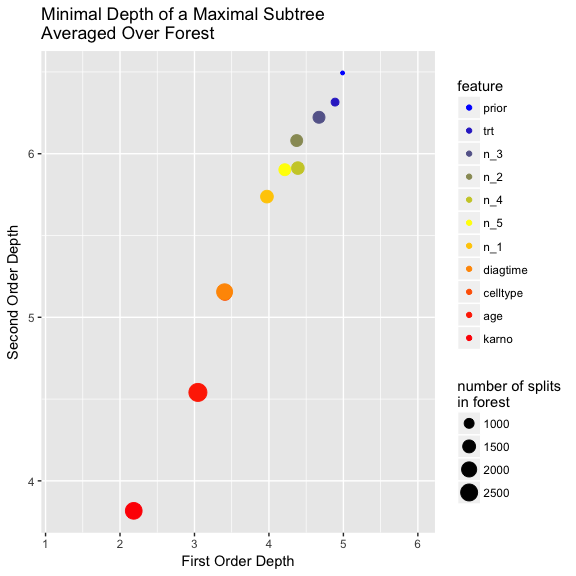In your figure, celltype probably outranked all the categorical noise variables of the same cardinality. This is great! It speaks to the power of the metric to differentiate between features of similar cardinality.

Citations: 1. Ishwaran, Hemant, et al. “High-dimensional variable selection for survival data.” Journal of the American Statistical Association 105.489 (2010): 205-217.